# Projectile Motion 9 2 Projectile motion http www

• Slides: 13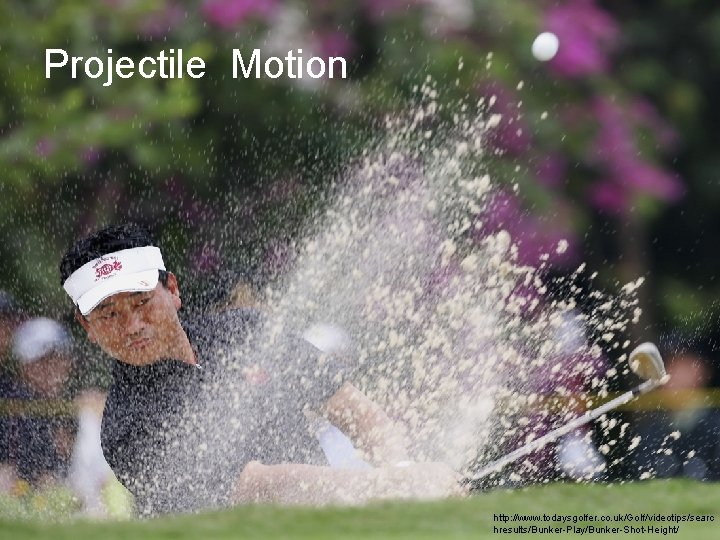Projectile Motion 9. 2 Projectile motion http: //www. todaysgolfer. co. uk/Golf/videotips/searc hresults/Bunker-Play/Bunker-Shot-Height/Projectile motion in every day life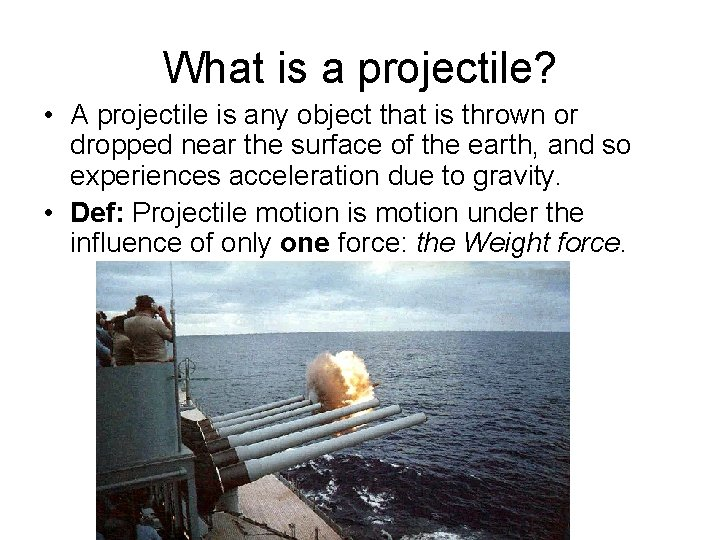What is a projectile? • A projectile is any object that is thrown or dropped near the surface of the earth, and so experiences acceleration due to gravity. • Def: Projectile motion is motion under the influence of only one force: the Weight force.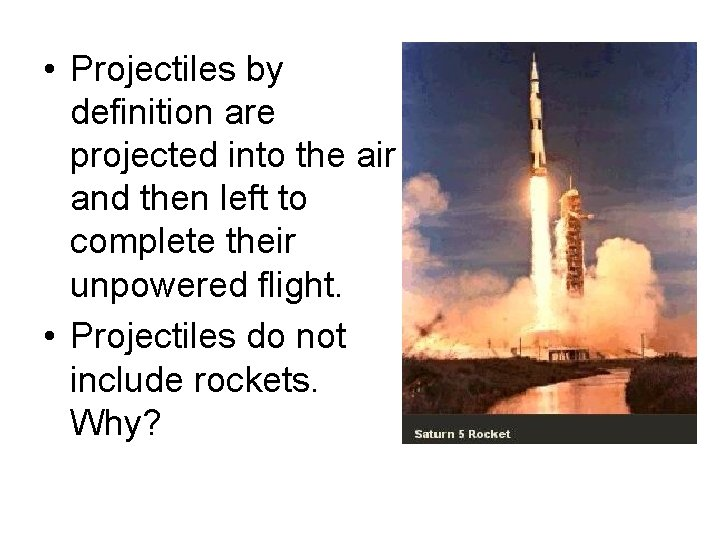• Projectiles by definition are projected into the air and then left to complete their unpowered flight. • Projectiles do not include rockets. Why?Forces on projectiles • Throughout flight the projectile is subject to only one force: gravity. • The projectile is subject to only one acceleration: g • Galileo’s analysis of projectile motion part 1: All objects fall at the same rate regardless of their mass. g is the same for all objectsFeather and hammer • If a feather and a hammer are dropped at the same time in the absence of air, they will hit the ground at the same time.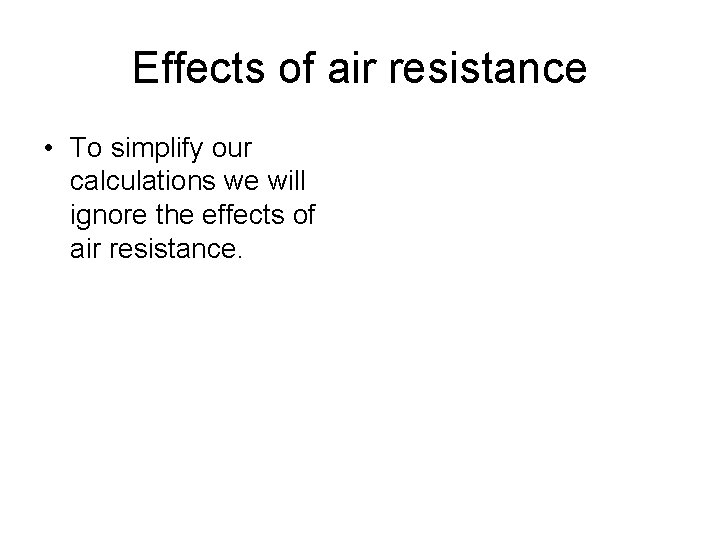Effects of air resistance • To simplify our calculations we will ignore the effects of air resistance.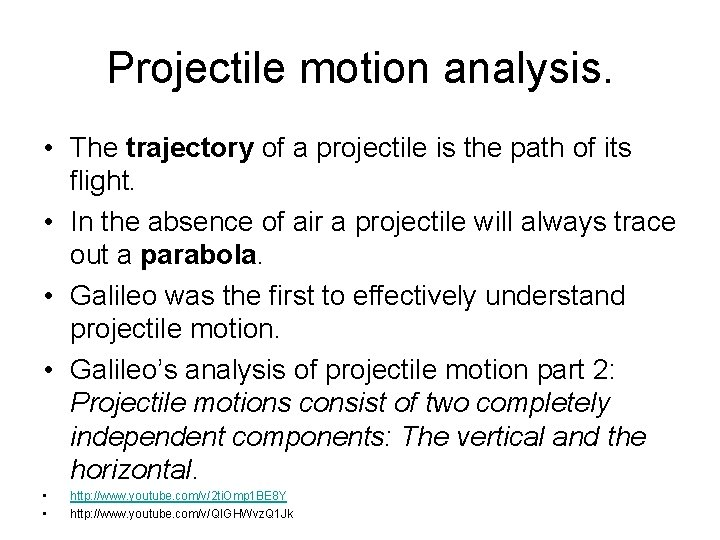Projectile motion analysis. • The trajectory of a projectile is the path of its flight. • In the absence of air a projectile will always trace out a parabola. • Galileo was the first to effectively understand projectile motion. • Galileo’s analysis of projectile motion part 2: Projectile motions consist of two completely independent components: The vertical and the horizontal. • • http: //www. youtube. com/v/2 ti. Omp 1 BE 8 Y http: //www. youtube. com/v/QIGHWvz. Q 1 Jk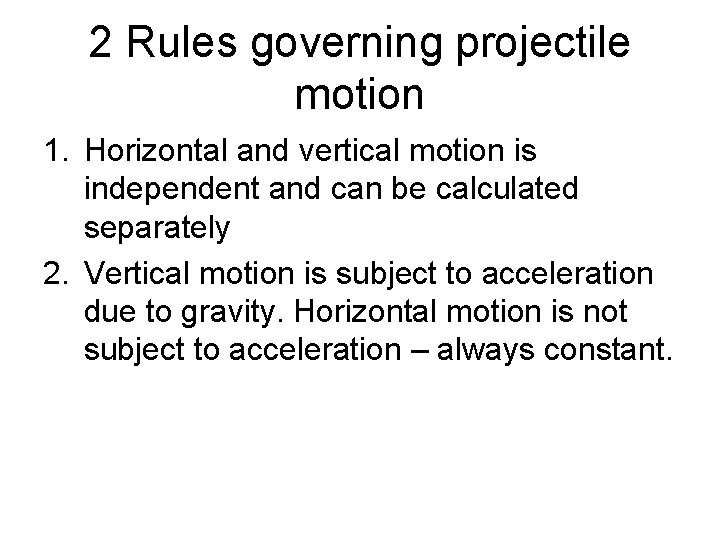2 Rules governing projectile motion 1. Horizontal and vertical motion is independent and can be calculated separately 2. Vertical motion is subject to acceleration due to gravity. Horizontal motion is not subject to acceleration – always constant.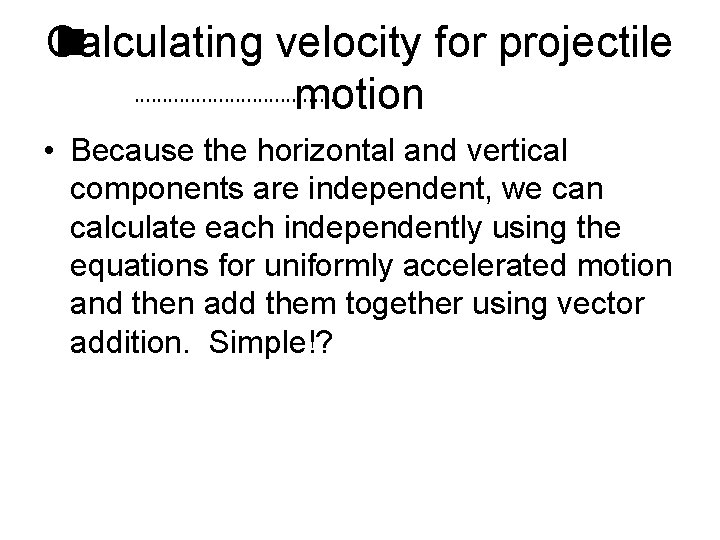Calculating velocity for projectile motion • Because the horizontal and vertical components are independent, we can calculate each independently using the equations for uniformly accelerated motion and then add them together using vector addition. Simple!?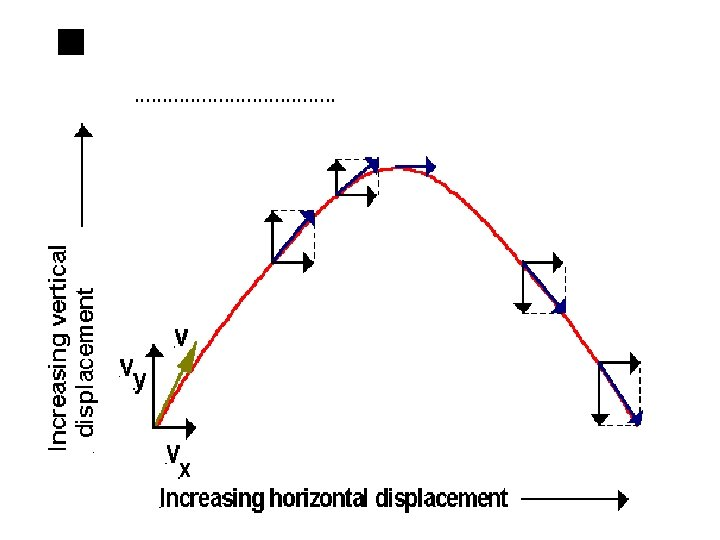Finding the Velocity from its Components If you know the horizontal and vertical velocity components, you can find the magnitude of the projectile's velocity and the projectile's direction of motion as follows: • Draw the horizontal and vertical velocity components (to scale) starting from a common point. • Draw 2 more sides to complete a rectangle. • The velocity of the projectile is the diagonal of the rectangle that starts at the point from which you drew the velocity components. • You can determine the magnitude of the velocity by measuring the scale drawing or using the Pythagorean Theorem. • You can determine the angle that the velocity makes with the horizontal (or vertical) by measuring the scale drawing with a protractor, or using trigonometry.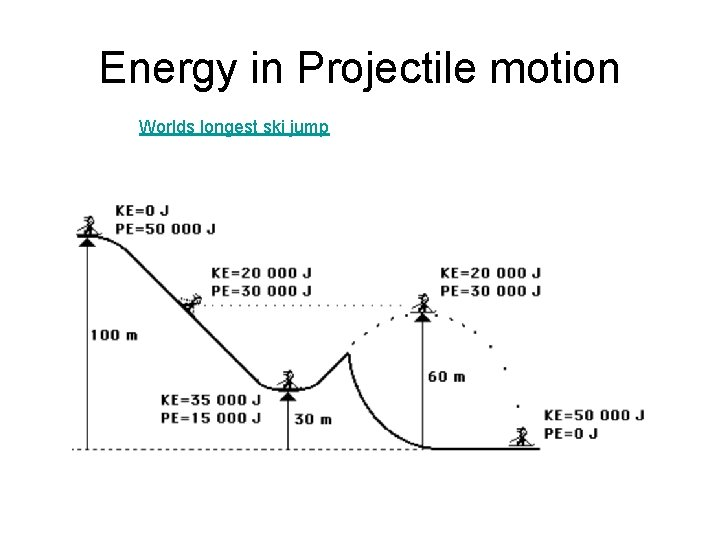Energy in Projectile motion Worlds longest ski jump